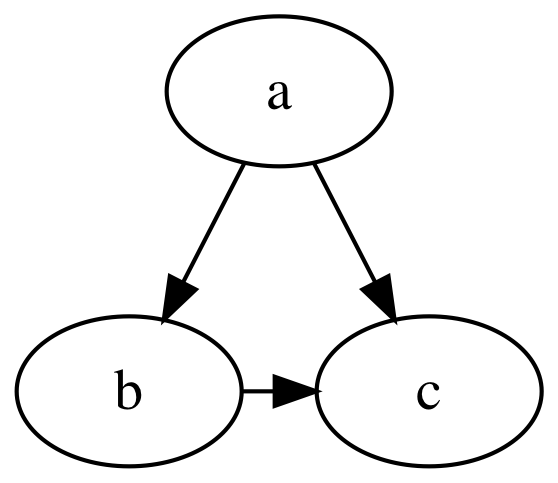## GraphViz

### master

A Swift package for working with GraphViz

# GraphViz

A Swift package for working with GraphViz.

## Requirements

• Swift 5.1+
• GraphViz (only for rendering)

## Usage

```import GraphViz

var graph = Graph(directed: true)

let a = Node("a"), b = Node("b"), c = Node("c")

graph.append(Edge(from: a, to: b))
graph.append(Edge(from: a, to: c))

var b_c = Edge(from: b, to: c)
b_c.constraint = false
graph.append(b_c)

// Produce DOT language representation
let dot = DOTEncoder().encode(graph)

// Render image using dot layout algorithm
let data = try! graph.render(using: .dot, to: .svg)
let svg = String(data: data, encoding: .utf8)!``````digraph {
a -> b
a -> c
b -> c [constraint=false]
}```

Note: The `render(using:to:)` method requires that the GraphViz binary corresponding to the specified layout algorithm is accessible from the current `\$PATH`.

### Using Function Builders, Custom Operators, and Fluent Attribute Setters

To use the following interface, add "GraphVizBuilder" to your package's dependencies and replace `import GraphViz` with `import GraphVizBuilder` as needed.

```import GraphVizBuilder

let graph = Graph(directed: true) {
"a" --> "b"
"a" --> "c"
("b" --> "c").constraint(false)
}```

Note: Swift 5.1 may require explicit typecast expressions in order to reconcile use of custom edge operators like `-->`. (`error: ambiguous reference to member '-->'`)

## Installation

### Swift Package Manager

Add the GraphViz package to your target dependencies in `Package.swift`:

```import PackageDescription

let package = Package(
name: "YourProject",
dependencies: [
.package(
url: "https://github.com/SwiftDocOrg/GraphViz",
from: "0.1.3"
),
]
)```

Add `GraphViz` as a dependency to your target(s):

```targets: [
.target(
name: "YourTarget",
dependencies: ["GraphViz"]),```

To render graphs to SVG, PNG, and other formats, you must have GraphViz executables (e.g. `dot`) installed on your system and accessible from `\$PATH`. You can install GraphViz from the command line:

``````# macOS
\$ brew install graphviz

# Linux (Ubuntu)
\$ sudo apt-get install graphviz
``````

MIT

## Contact

Mattt (@mattt)

### Description

• Swift Tools 5.1.0

### Dependencies

• None
Last updated: Wed Feb 01 2023 17:14:42 GMT-0500 (GMT-05:00)